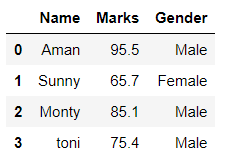# Difference between Pandas VS NumPy

## Difference between Pandas VS NumPy

Pandas is an open-source, BSD-licensed library written in Python Language. Pandas provide high performance, fast, easy to use data structures and data analysis tools for manipulating numeric data and time series. Pandas is built on the numpy library and written in languages like PythonCython, and C. In pandas, we can import data from various file formats like JSON, SQL, Microsoft Excel, etc.

Example:

 `# Importing pandas library` `import` `pandas as pd` ` ` `# Creating and initializing a nested list` `age ``=` `[[``'Aman'``, ``95.5``, ``"Male"``], [``'Sunny'``, ``65.7``, ``"Female"``],` `       ``[``'Monty'``, ``85.1``, ``"Male"``], [``'toni'``, ``75.4``, ``"Male"``]]` ` ` `# Creating a pandas dataframe` `df ``=` `pd.DataFrame(age, columns``=``[``'Name'``, ``'Marks'``, ``'Gender'``])` ` ` `# Printing dataframe` `df`

Output:NumpyIt is the fundamental library of python, used to perform scientific computing. It provides high-performance multidimensional arrays and tools to deal with them. A numpy array is a grid of values (of the same type) that are indexed by a tuple of positive integers, numpy arrays are fast, easy to understand, and give users the right to perform calculations across arrays.

Example:

 `# Importing Numpy package` `import` `numpy as np` ` ` `# Creating a 3-D numpy array using np.array()` `org_array ``=` `np.array([[``23``, ``46``, ``85``],` `                      ``[``43``, ``56``, ``99``],` `                      ``[``11``, ``34``, ``55``]])` ` ` `# Printing the Numpy array` `print``(org_array)`

Output:

```[[23 46 85]
[43 56 99]
[11 34 55]]
```

Table of Difference Between Pandas VS NumPy

Last Updated on March 17, 2022 by admin

## Python String isspace() MethodPython String isspace() Method

Python String isspace() Method Python String isspace() is a built-in method used for string handling. The isspace()

## Python program to get all unique combinations of two ListsPython program to get all unique combinations of two Lists

Python program to get all unique combinations of two Lists The combination is a mathematical

## Python – Get key from value in DictionaryPython – Get key from value in Dictionary

Python | Get key from value in Dictionary Let’s see how to get the key

## Django ORM – Inserting, Updating & Deleting DataDjango ORM – Inserting, Updating & Deleting Data

Django ORM – Inserting, Updating & Deleting Data Django lets us interact with its database

## How to compare two NumPy arrays?How to compare two NumPy arrays?

How to compare two NumPy arrays? This article focuses on the comparison done using NumPy

Difference between input() and sys.stdin.readline() Python is a widely used general-purpose language that can be

## Python – Check if all the values in a list that are greater than a given valuePython – Check if all the values in a list that are greater than a given value

Python | Check if all the values in a list that are greater than a

## Python – How to search for a string in text files?Python – How to search for a string in text files?

Python – How to search for a string in text files? In this article, we